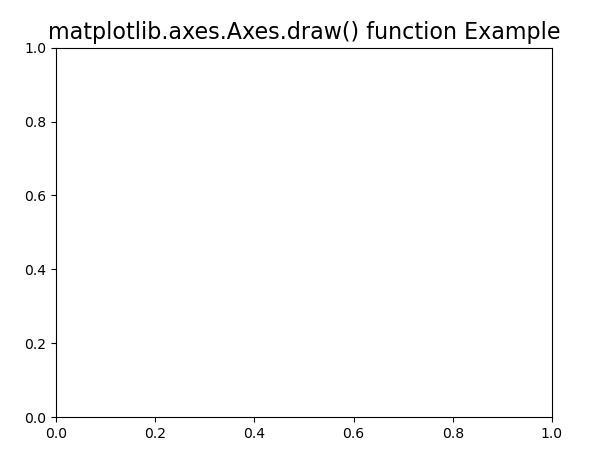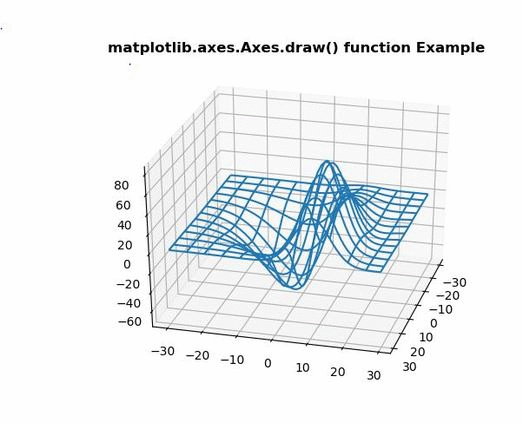# Matplotlib.axes.Axes.draw() in Python

Matplotlib is a library in Python and it is numerical – mathematical extension for NumPy library. The Axes Class contains most of the figure elements: Axis, Tick, Line2D, Text, Polygon, etc., and sets the coordinate system. And the instances of Axes supports callbacks through a callbacks attribute.

## matplotlib.axes.Axes.draw() Function

The Axes.draw() function in axes module of matplotlib library is used to draw everything.

Syntax: Axes.draw(self, renderer=None, inframe=False)

Parameters: This method accepts the following parameters.

• renderer: This parameter is the first parameter and its default value is None.
• inframe: This parameter contains the boolean value and its default value is false.

Returns: This method does not return any value.

Below examples illustrate the matplotlib.axes.Axes.draw() function in matplotlib.axes:

Example 1:

 `# Implementation of matplotlib function  ` `from` `mpl_toolkits.mplot3d ``import` `axes3d  ` `import` `matplotlib.pyplot as plt  ` `   `  ` `  `fig, ax ``=` `plt.subplots()  ` `   `  `def` `tellme(s):  ` `    ``ax.set_title(s, fontsize ``=` `16``)  ` `    ``fig.canvas.draw() ` `    ``renderer ``=` `fig.canvas.renderer ` `    ``ax.draw(renderer) ` ` `  `tellme(``'matplotlib.axes.Axes.draw() function Example'``)  ` `plt.show()  `

Output:Example 2:

 `# Implementation of matplotlib function  ` `from` `mpl_toolkits.mplot3d ``import` `axes3d  ` `import` `matplotlib.pyplot as plt  ` `   `  ` `  `fig ``=` `plt.figure()  ` `ax ``=` `fig.add_subplot(``111``, projection ``=``'3d'``)  ` `   `  `X, Y, Z ``=` `axes3d.get_test_data(``0.1``)  ` `ax.plot_wireframe(X, Y, Z, rstride ``=` `5``,   ` `                  ``cstride ``=` `5``)  ` `   `  `for` `angle ``in` `range``(``0``, ``90``):  ` `    ``ax.view_init(``30``, angle) ` `    ``fig.canvas.draw() ` `    ``renderer ``=` `fig.canvas.renderer ` `    ``ax.draw(renderer)  ` `    ``plt.pause(.``001``)  ` `    ``ax.set_title('matplotlib.axes.Axes.draw()\ ` `    ``function Example', fontweight ``=``"bold"``)  `

Output:Whether you're preparing for your first job interview or aiming to upskill in this ever-evolving tech landscape, GeeksforGeeks Courses are your key to success. We provide top-quality content at affordable prices, all geared towards accelerating your growth in a time-bound manner. Join the millions we've already empowered, and we're here to do the same for you. Don't miss out - check it out now!

Previous
Next